Next: 3.10.2 Estimates Based on Up: 3.10 Exposure Time Estimates Previous: 3.10 Exposure Time Estimates

3.10.1 Estimating Exposure Times from Known UV Fluxes

The procedures for determining exposure times from known or estimated UV fluxes are given below. Low-dispersion exposure times are scaled from the corresponding estimates for high dispersion.

3.10.1.1 High-Dispersion Large Aperture Exposures

A.
Estimating Peak Blaze Continuum Exposures Approximate IUE exposure times in seconds may be determined using the following expression: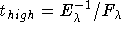for large aperture, high dispersion

whereis related to the inverse sensitivity function (see Figure 3.5), and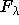is the flux in erg cm¯² s¯¹ Å¯¹ for a continuum point source or erg cm¯² s¯¹ Å¯¹ (10 arcsecond²)¯¹ for an extended source.

Estimates ofin erg cm¯² s¯¹ Å¯¹ may be obtained from published results of OAO-2 (e.g. Code and Meade 1979), Copernicus (e.g. Snow and Jenkins 1977), TD-1 (e.g. Jamar et al. 1976, or Thompson et al. 1978) and ANS satellites (Wesselius et al. 1982).

The values ofgiven in Figure 3.5 are based on the stellar flux calibration used by OAO-2 and Copernicus. TD1 and ANS fluxes are based on different calibrations. Multiply TD1 and ANS fluxes by the factors given in Table 3.4 to convert to the OAO-2 scale.

 Wavelength TD1 ANS E(-V)/E(B-V) 1380Å 1.35 -- 5.44 1550Å 1.37 1.35 5.04 1800Å 1.15 1.14 4.65 2200Å 1.02 0.93 6.55 2500Å 1.13 1.02 4.19 2700Å 1.32 -- 3.24 3300Å -- 1.09 1.95 Values interpolated from those of Savage and Mathis (1979).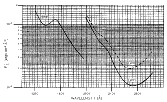Figure 3.5: IUE inverse sensitivity functions. (Note: LWR sensitivity curve is for the 4.5 kV configuration and includes the sensitivity degradation as of mid-1986.)

Be sure to correct for interstellar extinction when estimating fluxes for reddened stars from those for unreddened stars. Interpolated average normalized interstellar extinction for the TD1 and ANS fluxes are also included in Table 3.4.

B.
Estimating Off-Peak Blaze Exposures Features which are located off the peak of the echelle blaze will require longer exposure times to bring them up to the optimum level. For example, if an exposure is optimized for the 2800Å Mg II absorption line, the adjacent continuum in the center of the order (2780-2790Å) may be overexposed.=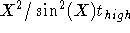where

X =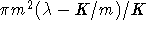K = 137,725 for the SWP camera
K = 231,150 for the LWR camera
K = 230,701 for the LWP camera

and

m = the order number, where m = INTEGER[K/+ 0.5]

3.10.1.2 High-Dispersion Small Aperture Exposures

For small aperture spectra, multiply large aperture exposure times by 1.9 or 2.0 for the short- and long-wavelength spectrograph, respectively, to compensate for the reduced throughput of the small apertures. Note that the relative throughput of the small aperture is variable (50% ±25) and so the small aperture is not appropriate for absolute flux determination.

3.10.1.3 Low-Dispersion Large Aperture Exposures

Low dispersion exposure time estimates are derived from scaling the high dispersion estimates, as described below.

A.
Continuum Point Source The following relations provide the factors to scale exposures times between low and high dispersion for point source, large aperture spectra:

t(low) = t(high)/87 = (/)/87 for SWP
t(low) = t(high)/70 for LWP
t(low) = t(high)/65 for LWR

B.
Emission Point Source The ratio of high-to-low dispersion exposure times for emission line sources will depend on the width of the line in question. For emission line sources this ratio will be smaller by a factor of W/6 than that for continuum sources where W is the FWHM of the emission line in Angstroms. Typically W/6 is 0.3 to 0.5 so that, for example, the ratio for the LWP would drop from 70 to range of 21 to 35.
C.
Trailed (Widened) Spectra (see Section 4.8)

3.10.1.4 Low-Dispersion Small Aperture Exposures

Refer to Section 3.10.1.2.Next: 3.10.2 Estimates Based on Up: 3.10 Exposure Time Estimates Previous: 3.10 Exposure Time Estimates

Last updated: 24 July 1997
jrc